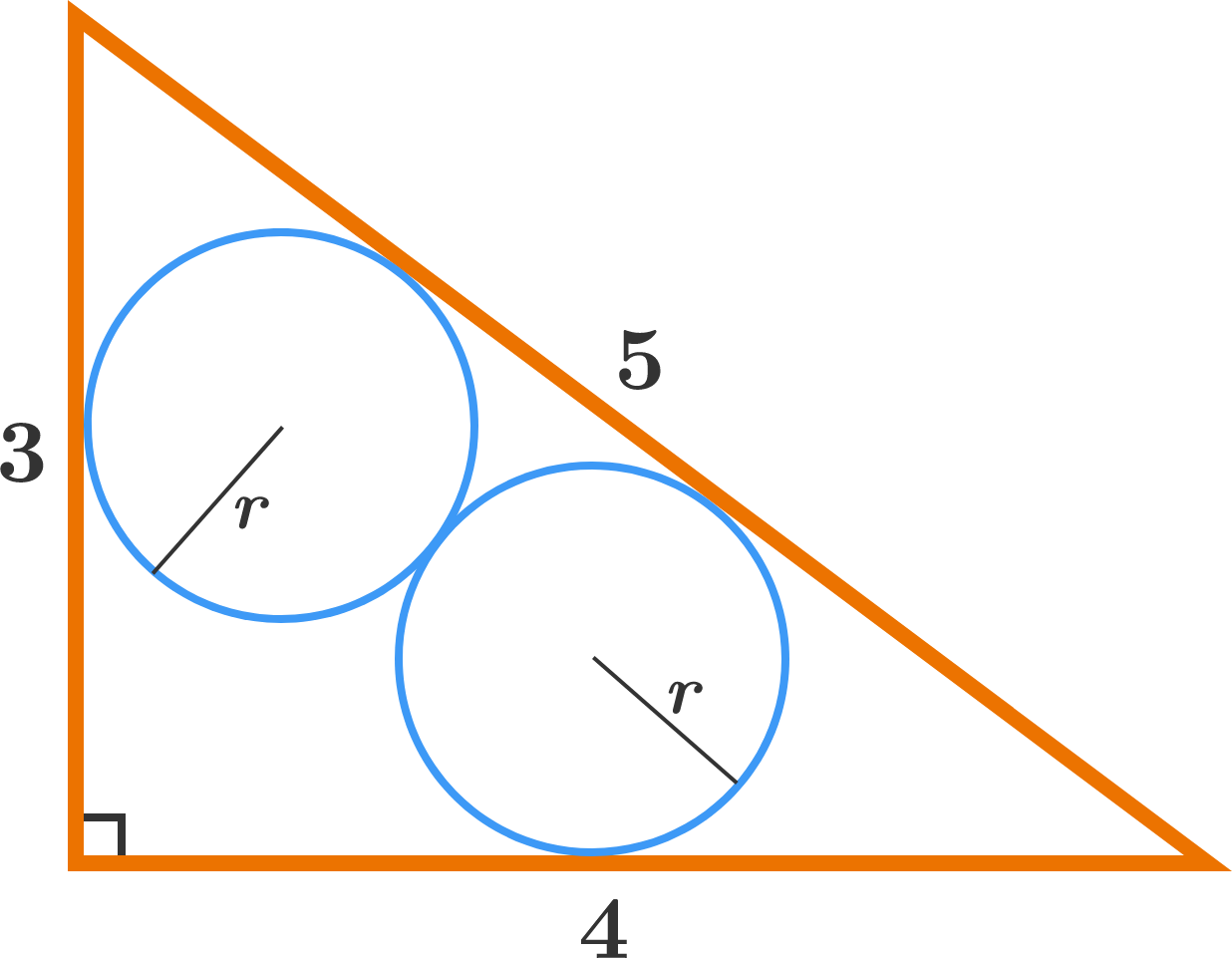# Twins in the Trigon

Geometry Level 4The figure above shows two equal circles of radius $r$ that are inscribed inside a triangle with side lengths 3, 4, 5.

The two circles share 1 tangent point.

If $r$ can be expressed as $\frac AB$, where $A$ and $B$ are coprime positive integers, find $A+B$.

×